# Return every n-th item using VBA in Microsoft Excel

In case you are wondering how someone can find every nth item in a column. In this article we will learn how to return every n-th item from a list. We will use VBA code to find the result.

Question: I want a macro code to extract every nth value from a column. I am trying to pull values from one sheet / column into another sheet / column. I tried using many functions like OFFSET but not able to figure out the formula that can give the result.

Let us take an example of 100 values in column A & we want to find out every 10th value in column B

The sample values starting from cell A1 equal to 1 to cell A100 equal to 100; following is the snapshot of data in column A: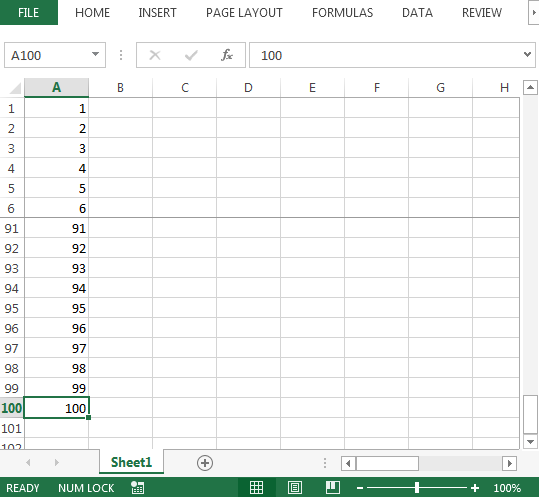To get result, we need to follow the below steps to launch VB editor

• Click on Developer tab
• From Code group, select Visual Basic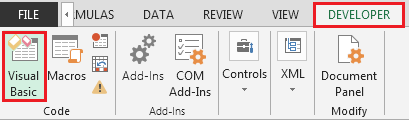• Copy the below code in the standard module

```Function INDEXN(InputRange As Range, N As Integer) As Variant
' returns every N-th item from InputRange' --- Comment
' select the desired target range for the function and' --- Comment
' enter as an array function with Ctrl+Shift+Enter.' --- Comment
Dim ItemList() As Variant, c As Range, i As Long, iCount As Long
i = 0
iCount = 0
ReDim ItemList(1 To InputRange.Cells.Count \ N)
For Each c In InputRange
i = i + 1
If i Mod N = 0 Then
iCount = iCount + 1
On Error Resume Next
ItemList(iCount) = c.Value
On Error GoTo 0
End If
Next c
INDEXN = ItemList
If InputRange.Rows.Count >= InputRange.Columns.Count Then
INDEXN = Application.WorksheetFunction.Transpose(INDEXN)
End If
Erase ItemList
End Function

```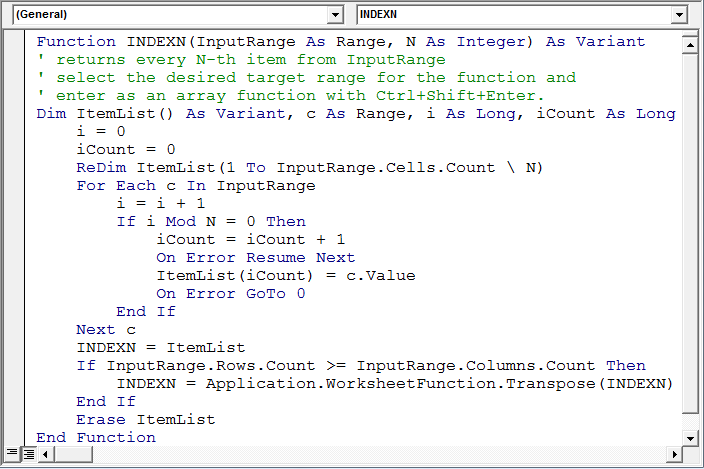• To get every nth item in range B1:B10, the formula is
• {=INDEXN(\$A\$1:\$A\$100, 10)}

Note: this is an array formula; hence, Ctrl + Shift + End keys must be pressed together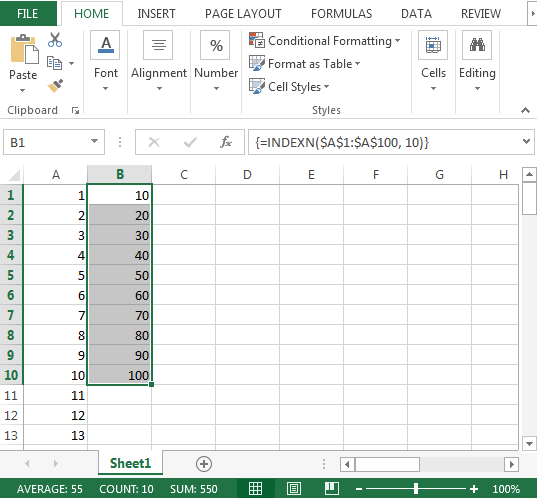• To get every 7th value from range A1:A100; we will change the last argument from 10 to 7 & formula will be
• {=INDEXN(\$A\$1:\$A\$100, 7)}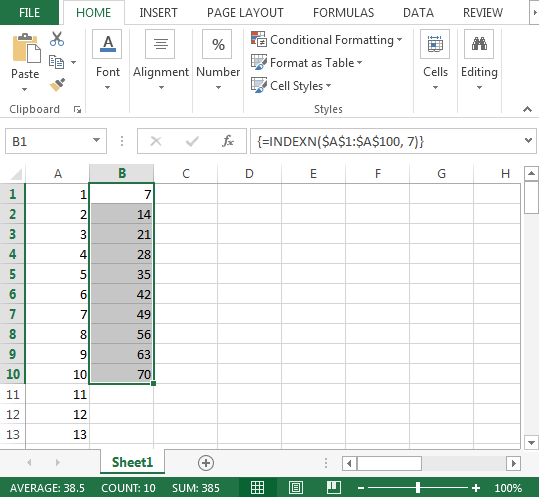In this way, we can return every n-th item from one sheet to master sheet.

• The formula in other sheet will contain sheet reference
• {=INDEXN(Sheet1!\$A\$1:\$A\$100, 10)}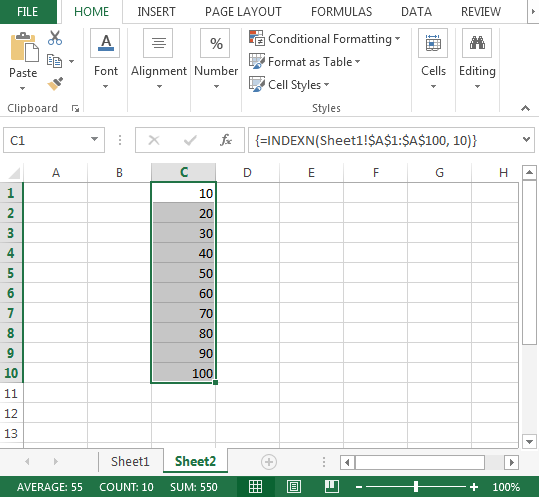Conclusion: With the help of above User-Defined Function, we can have flexibility to find out every nth item from one sheet to another.If you liked our blogs, share it with your friends on Facebook. And also you can follow us on Twitter and Facebook. We would love to hear from you, do let us know how we can improve, complement or innovate our work and make it better for you. Write us at info@exceltip.com

1.